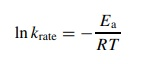# Determining Activation Energies

| Home | | Organic Chemistry |

## Chapter: Organic Chemistry : Mechanisms of Organic Reactions

By using the above methods, the rate constants for most organic reactions can be obtained.

DETERMINING ACTIVATION ENERGIES

By using the above methods, the rate constants for most organic reactions can be obtained. Rate constants, by virtue of the fact that they relate directly to the passage of reactants over the barrier of the rate-determining step, can be used to probe the energy and structure of the activated complex. The energy of the activated complex corresponds to the height of the activation barrier for the rate-determining step. The barrier height can be calculated by the Arrhenius equationIn krate = - Ea/RT

where krate is the rate constant of a reaction, R is the ideal gas constant, and T is the absolute temperature. The Arrhenius activation energy Ea is determined from plots of ln k versus 1/T at various temperatures and largely corresponds to the enthalpy of activation since Ea = ΔH± + RT. The enthalpy and entropy of activation and hence the free energy of activation are determined by the Eyring equationwhere k is Boltzmann’s constant and h Planck’s constant. Plots of ln(krate/T ) versus 1/T give straight lines with slope – ΔH±/R and intercept ΔS ± /R − ln(k/ h). Each can be numerically evaluated to give ΔH±, ΔS±, and finally ΔG± by ΔG± = ΔH± − TΔS±

Contact Us, Privacy Policy, Terms and Compliant, DMCA Policy and Compliant

TH 2019 - 2022 pharmacy180.com; Developed by Therithal info.